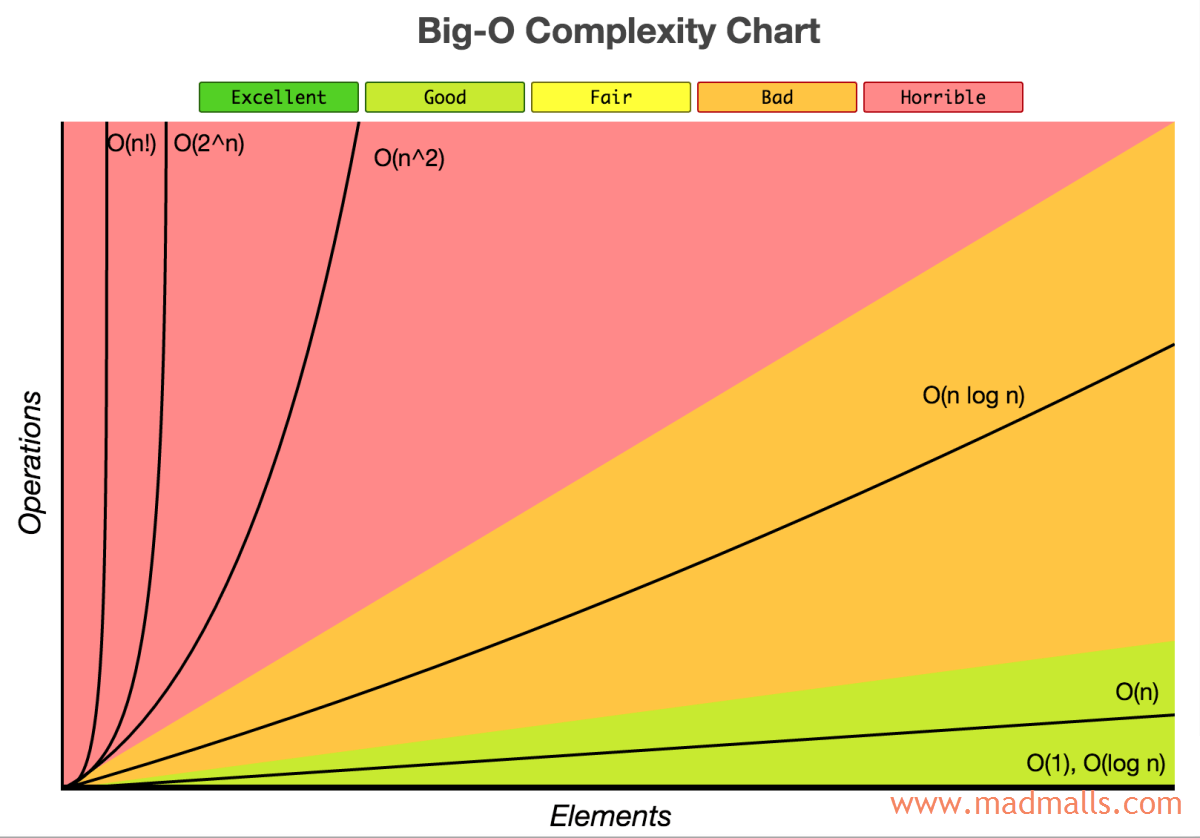# 算法概述

• /
• /
• 0
• 2609 次阅读

Synopsis: 算法是设计良好的可计算的过程，它把某个值或某些值作为输入并产生某个值或某些值作为输出。通常设计一个解决问题的算法是很容易的，但如果这个算法很慢，就要重新设计了。因为算法运行的速度取决于它运行的环境以及实现的细节，计算机科学家们倾向于把运行时间以输入的大小来表示，比如O(n)

# 1. 特征

An algorithm is a sequence of unambiguous instructions used for solving a problem, which can be implemented (as a program) on a computer

`算法`是解决问题的方法，可以用不同的计算机语言去实现该算法，重要的是算法的思想！• `输入（Input）`： 一个算法必须有0个或0个以上输入量。Every algorithm must take zero or more number of input values from external.
• `输出（Output）`： 一个算法应有1个或1个以上输出量，输出量是算法计算的结果。Every algorithm must produce an output as result.
• `明确性（Definiteness）`： 算法的描述必须无歧义，算法中的每个语句/指令都必须清晰明确（只有一种解释）。Every statement/instruction in an algorithm must be clear and unambiguous (only one interpretation)
• `有限性（Finiteness）`： 算法必须在有限个步骤内完成任务。For all different cases, the algorithm must produce result within a finite number of steps.
• `有效性（Effectiveness）`： 又称`可行性`，算法中描述的每个操作，都可以通过执行有限次的基本运算来实现。Every instruction must be basic enough to be carried out and it also must be feasible.

# 2. 算法的性能

## 2.1 时间复杂度

• `O(1)`： 常数阶（不会随 n 的大小而改变）
• `O(log n)`： 对数阶
• `O(n)`： 线性阶
• `O(n log n)`： 线性对数阶
• `O(n²)`： 平方阶
• `O(n³)`： 立方阶
• `O(2^n)`： 指数阶
• `O(n!)`： 阶乘阶## 2.2 空间复杂度

1. `Instruction Space`： It is the amount of memory used to store compiled version of instructions.
2. `Environmental Stack`： It is the amount of memory used to store information of partially executed functions at the time of function call.
3. `Data Space`： It is the amount of memory used to store all the variables and constants.

`时间复杂度`相比，`空间复杂度`的分析要简单得多，我们只考虑`数据空间(Data Space)`并忽略指令空间以及环境堆栈，这意味着我们只计算存储`变量``常量``结构`等所需的内存

## 2.3 示例

```import time
from functools import wraps

def timethis(func):  # 装饰器，输出被装饰的函数的运行时间
@wraps(func)
def wrapper(*args, **kwargs):
start = time.process_time()
r = func(*args, **kwargs)
end = time.process_time()
print('Function {}() in model {} cost: {} seconds'.format(func.__name__, func.__module__, end - start))
return r
return wrapper
```

### (1) 算法1

```from timethis import timethis

@timethis
def combination():  # 算法1，3层for循环，时间复杂度为O(n^3)
for a in range(1001):
for b in range(1001):
for c in range(1001):
if a**2 + b**2 == c**2 and a+b+c == 1000:
print("a, b, c: %d, %d, %d" % (a, b, c))

if __name__ == '__main__':
combination()

# Output:
a, b, c: 0, 500, 500
a, b, c: 200, 375, 425
a, b, c: 375, 200, 425
a, b, c: 500, 0, 500
Function combination() in model __main__ cost: 1137.25 seconds
```

### (2) 算法2

```from timethis import timethis

@timethis
def combination():  # 算法2，2层for循环，时间复杂度为O(n^2)
for a in range(1001):
for b in range(1001-a):
c = 1000 - a - b
if a**2 + b**2 == c**2:
print("a, b, c: %d, %d, %d" % (a, b, c))

if __name__ == '__main__':
combination()

# Output:
a, b, c: 0, 500, 500
a, b, c: 200, 375, 425
a, b, c: 375, 200, 425
a, b, c: 500, 0, 500
Function combination() in model __main__ cost: 0.65625 seconds
```

```from timethis import timethis

@timethis
def combination():  # 算法2，2层for循环，时间复杂度为O(n^2)
for a in range(10001):
for b in range(10001-a):
c = 10000 - a - b
if a**2 + b**2 == c**2:
print("a, b, c: %d, %d, %d" % (a, b, c))

if __name__ == '__main__':
combination()

# Output:
a, b, c: 0, 5000, 5000
a, b, c: 2000, 3750, 4250
a, b, c: 3750, 2000, 4250
a, b, c: 5000, 0, 5000
Function combination() in model __main__ cost: 62.90625 seconds
```

# 3. 排序算法

• `[(3, 1), (3, 7), (4, 1), （5, 6）]`，其中元素`(3, 1)``(3, 7)`维持它们排序前的次序，则称该排序算法是`稳定`
• `[(3, 7), (3, 1), (4, 1), （5, 6）]`，其中元素`(3, 1)``(3, 7)`的次序与它们排序前不同，则称该排序算法是`不稳定`

# 4. 查找算法

## 作者